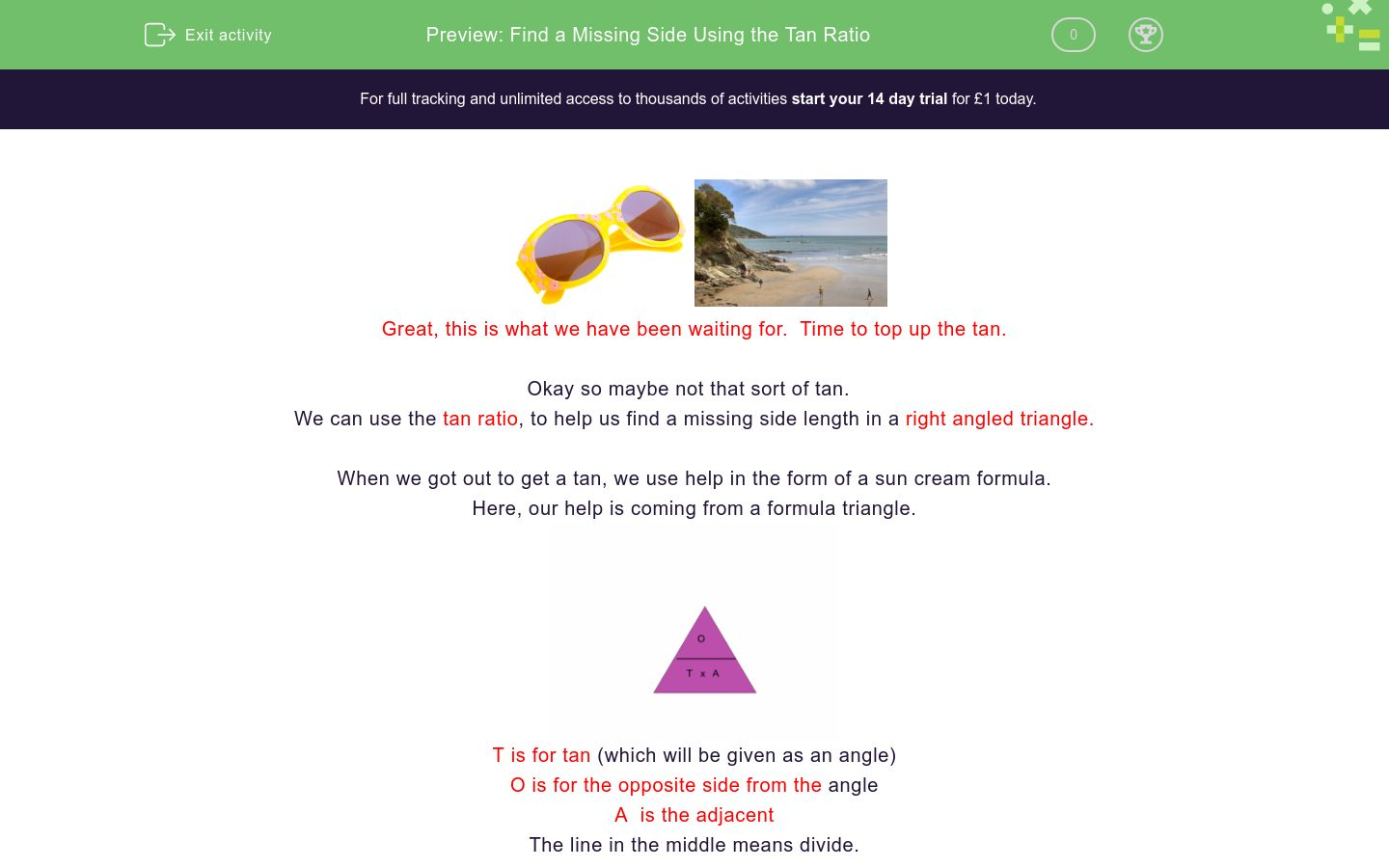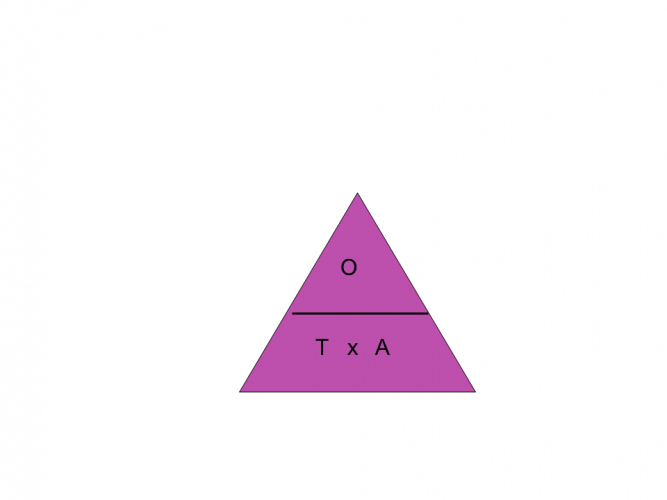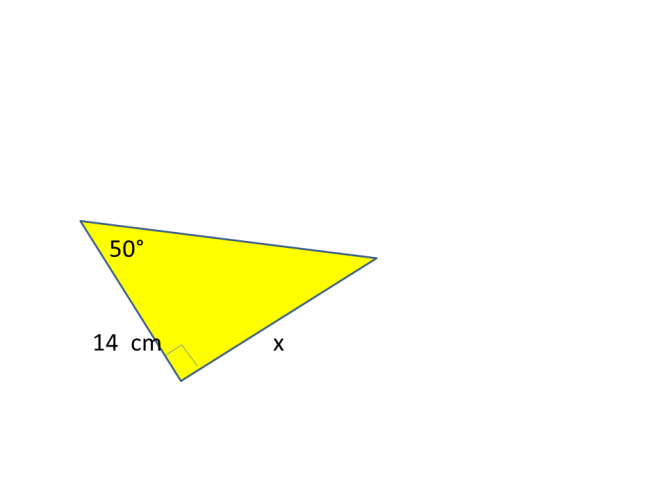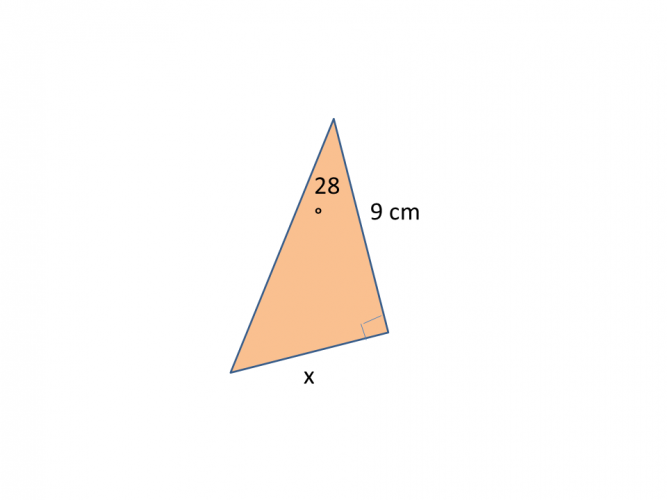# Find a Missing Side Using the Tan Ratio

In this worksheet, students will use the Tan ratio to find the missing side of a triangle.Key stage:  KS 4

GCSE Subjects:   Maths

GCSE Boards:   AQA, Eduqas, Pearson Edexcel, OCR

Curriculum topic:   Geometry and Measures, Mensuration

Curriculum subtopic:   Properties and Constructions, Mensuration and Calculation, Triangle Mensuration

Difficulty level:### QUESTION 1 of 10Great, this is what we have been waiting for.  Time to top up the tan.

Okay so maybe not that sort of tan.

We can use the tan ratio, to help us find a missing side length in a right angled triangle.

When we got out to get a tan, we use help in the form of a sun cream formula.

Here, our help is coming from a formula triangle.T is for tan (which will be given as an angle)

O is for the opposite side from the angle

The line in the middle means divide.

To use this triangle we cover up what side it is we want to find and we are left with a formula to follow.

Who would have thought formulas could be so helpful.If we want to find the adjacent, cover up the A.  The formula we are left with is opposite ÷ tan(angle)If we want to find the opposite cover up the O.  The formula we are left with is Tan(angle) x Adjacent.Let's give it a go.

You will need a scientific calculator for this.  Make sure it is set to degrees.

Example 11. Label the triangle

2. Find the two sides you want.  You want to find x, so we want O, and the only other side you have to help is the A.

3. Look at your formula triangle, cover up the side you want to find which is O. (see above)

4.  We are left with tan47 x 7

5. In your calculator type in  tan 47 x 7

Example 22. Find the sides you want

3.  You should have discovered you wanted O and A

4. Use your formula triangle and cover up A the side you want to find

5. The formula you are left with is opposite ÷ tan 32

6. 15 ÷ tan32 = 24.00 cm correct to 2 decimal places

How tantastic is that?Find the length marked x.

Answer has been rounded to 2 decimal places

12.50 cm

11.00 cm

11.12 cm

12.65 cmFind the value of xtan x adjacent opposite divided by tan 12 cm 14 cm 16 cm The formula I need is The value of x istan x adjacent opposite divided by tan 12 cm 14 cm 16 cm The formula I need is The value of x isFind the length of x

42.25 cm

31.87 cm

32.34 cm

29.55 cmtan x adjacent opposite divided by tan 10.14 cm 8.43 cm 6.11 cm The formula I need is the value of x istan x adjacent opposite divided by tan 10.14 cm 8.43 cm 6.11 cm The formula I need is the value of x isFind the length of x.Find the value of x.

Answer has been rounded to 2 decimal places (2 d.p)

4.47 cm

5.42 cm

4.79 cm

5.24 cm

• Question 1Find the length marked x.

Answer has been rounded to 2 decimal places

11.00 cm
EDDIE SAYS
Labelling your triangle correctly can be tantalisingly tricky. You should have discovered that you needed the opposite so our formula is tan45 x 11 tan 45 x 11= 11.00 cm (to 2 d.p)
• Question 2Find the value of x

12.2
EDDIE SAYS
How are you going with the labelling of the triangle? It can get tricky I know. You should have discovered that you needed the tan x the adjacent tan 62 x 6.5 = 12.22 (12.2 to 1 d.p)
• Question 3tan x adjacent opposite divided by tan 12 cm 14 cm 16 cm The formula I need is The value of x is
EDDIE SAYS
I hope you are not getting tan(gled) up labelling the triangle. Keep practicing until it becomes second nature. You should have discovered you needed opposite and the tangent. 12 ÷ tan45 = 12cm
• Question 4EDDIE SAYS
Using our formula triangle shows us that we need to find the opposite side, so the formula was tan33 x 42 = 27.28 cm
• Question 5Find the length of x

32.34 cm
EDDIE SAYS
Are you getting used to this now? We want the adjacent side so the formula is opposite ÷ tan33 21 ÷ tan33 = 32.34 cm to (2 d.p). When finding a side we always need the angle, it is just a case of deciding if we multiply or divide with it.
• Question 616.68
EDDIE SAYS
I hope your trigonometry teacher is a good one, and they don't always go off on a tangent. Keeping on track gives us tan x adjacent tan50 x 14 = = 16.68 cm (to 2 d.p)
• Question 7tan x adjacent opposite divided by tan 10.14 cm 8.43 cm 6.11 cm The formula I need is the value of x is
EDDIE SAYS
I hope you are getting the hang of this now and not having tantrums. We can see here that we need.. 12 ÷ tan63 = 6.11 cm
• Question 8EDDIE SAYS
Calculators rock. The only thing you have to be careful of, is rounding to the correct number of decimal places. tan33 x 8.5 = 5.52 cm (to 2 d.p)
• Question 9Find the length of x.

14.40
EDDIE SAYS
Nearly there. I hope you haven't found this to tri-ing!! Using the formula triangle at the beginning is tantalisingly magic. opposite ÷ tan32 9 ÷ tan32 = 14.40 (to 2 d.p)
• Question 10Find the value of x.

Answer has been rounded to 2 decimal places (2 d.p)

4.79 cm
EDDIE SAYS
And finally. One final check though tan28 x 9 = 4.79 cm (to 2 d.p) I hope everything is tantastic.
---- OR ----

Sign up for a £1 trial so you can track and measure your child's progress on this activity.

### What is EdPlace?

We're your National Curriculum aligned online education content provider helping each child succeed in English, maths and science from year 1 to GCSE. With an EdPlace account you’ll be able to track and measure progress, helping each child achieve their best. We build confidence and attainment by personalising each child’s learning at a level that suits them.

Get started